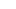# CAT Quant: Solving Advanced Probability-Based Questions

(Photo: Lydia)

In my previous post on probability, we talked about the basics of the topic and I honestly believe that that much should be enough for most CAT applicants. But there is a finite probability that a slightly more advanced question on probability might be asked in CAT. The probability that a more advanced question on probability will be asked in XAT is a littler higher than the probability of it being asked in CAT. Confused? Don’t be – I am just being geeky here.

In the previous post, we had talked about discrete variables whereas today we will talk about continuous variables. The most common type of discrete variable is a count, in which case it must be a whole number. Examples include family size, number of road deaths and number of cigarettes smoked per day, result on throw of a dice. Continuous variables are defined on a continuous scale. Examples include length, weight, etc.

Let us look at some of the classic problems on probability with continuous variables and how to solve them.

Broken Stick Problem

If you break a stick randomly in two places, what is the probability that you can form a triangle from the three pieces?

To solve this, let us consider the length of the stick as 1 and the two points that it is broken at as ‘x’ and ‘y’ where xSo, the length of the three parts will be x, y – x and 1 – y

Let us represent this on a graphThe point P, which gives us the exact points at which the stick was broken will lie inside a square of side 1.

For the three parts to form a triangle, they should satisfy the triangle criteria that the sum of two sides is greater than the third side.

x + (y – x) > (1 – y)

=> y > ½

x + (1 – y) > (y – x)

=> 2y – 2x

=> y – x

(y – x) + (1 – y) > x

=> x

If we apply these conditions on the graph we will get,To meet the criteria, P(x,y) has to lie in the triangle shown above.

The probability of that happening = Area of the triangle / total area = 1/8

The exact same pattern will be repeated when x > y, and we will get the probability again as 1/8 in that case.

Required probability = 1/8 + 1/8 = ¼

Minimum Length Problem

Two points A and B are selected at random on a segment of length 2 cm. What is the probability that the length of the line AB > 1 ?

Apply the exact same idea as discussed in the broken stick problem. Our basic graph would look like:As you can seem the blue line is used to indicate that A = B.

We want the probability that the distance between A and B is greater than 1

=> The distance from the line A = B should be at least one unit

Using this restriction, we would get the graph asSo, our point P can lie in the either the triangle on the top-left or in the triangle on the bottom right.

Required probability = Sum of the areas of the triangle / Total area

= (0.5 + 0.5)/4 = ¼

Time Related Problem

Two ISB alumni decide to meet at Café Linger On between 9:30 am and 10:30 am. They agree that the person who arrives first at the cafe would wait for exactly 15 minutes for the other. If each of them arrives at a random time between 9:30 AM and 10:30 AM . What is the probability that the meeting takes place?

This can be solved exactly with the same concept of ‘Minimum Length Problem’.

The time period in which they can arrive is 1 hour and they have to be within 15 minutes of each other or 0.25 units of each other.

=> The distance from the line A = B should be less than 0.25 units

Required probability = 1 – Unfavorable probability

= 1 – (Sum of the areas of the two triangles / Total Area)

= 1 – 2*(½*¾*¾) = 7/16

Ravi Handa has taught Quantitative Aptitude at various coaching institutes for seven years. An alumnus of IIT Kharagpur where he studied a dual-degree in computer science, he currently runs an Online CAT Coaching and CAT Preparation Course and the website Handa Ka Funda.